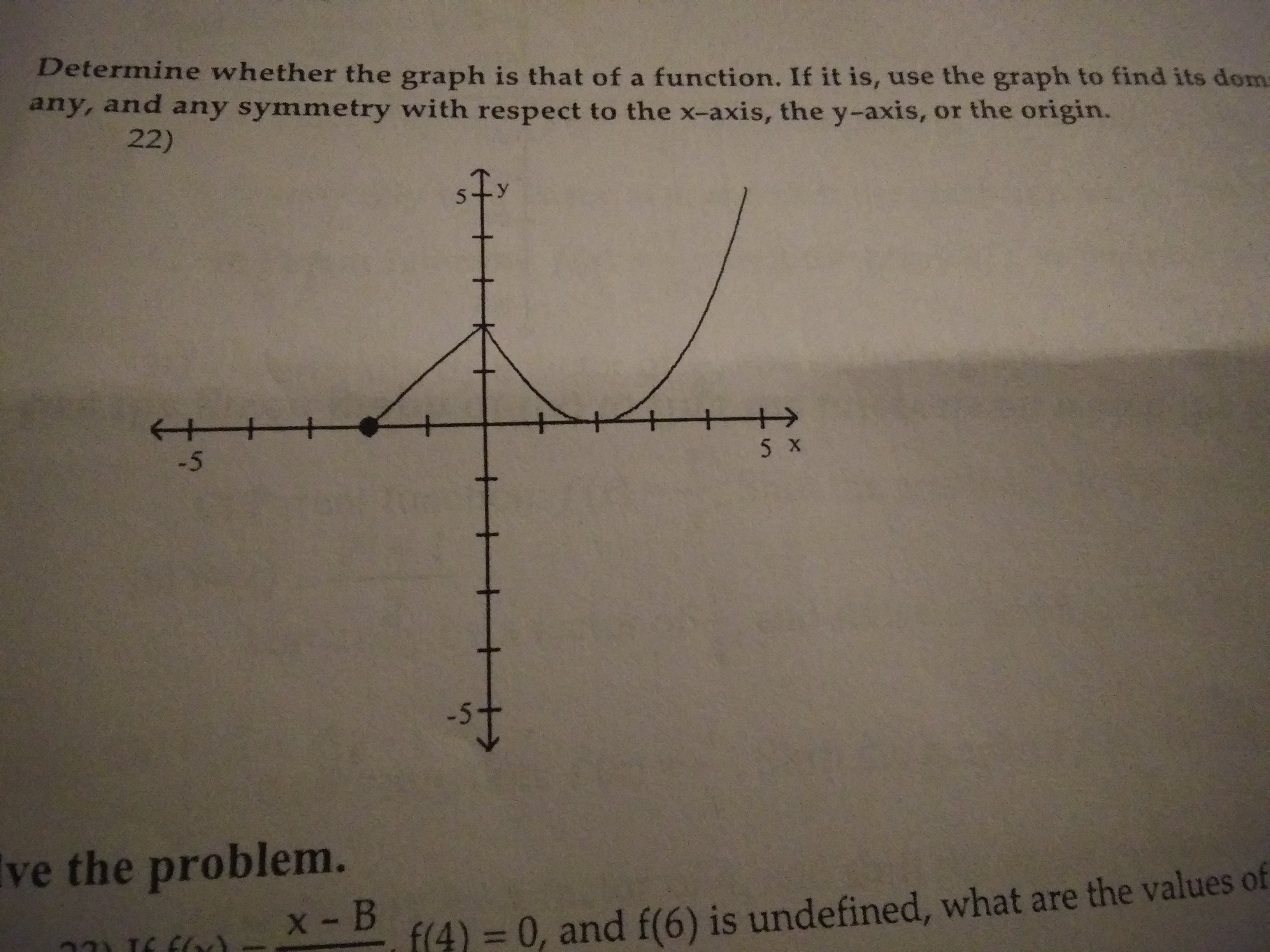# Determine whether the graph is that of a function. If it is, use the graph to find its domsany, and any symmetry with respect to the x-axis, the y-axis, or the origin.22)-5Ive the problem.x- Bf(4) 3D0, and f(6) is undefined, what are the values of-5

Question
4 views

Determine whether the graph is that of a function. If it is, use the graoh to find its domain and range, the intercepts, if any symmetry with respect to the x-axis or the origin.help_outlineImage TranscriptioncloseDetermine whether the graph is that of a function. If it is, use the graph to find its doms any, and any symmetry with respect to the x-axis, the y-axis, or the origin. 22) -5 Ive the problem. x- B f(4) 3D0, and f(6) is undefined, what are the values of -5 fullscreen
check_circle

Step 1

Consider the provided graph,

From the vertical line test, if the vertical line intersect the graph at only one point then the graph is a function.

Now, in the given grap...

### Want to see the full answer?

See Solution

#### Want to see this answer and more?

Solutions are written by subject experts who are available 24/7. Questions are typically answered within 1 hour.*

See Solution
*Response times may vary by subject and question.
Tagged in

### Other